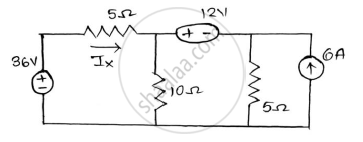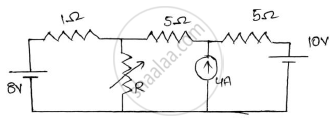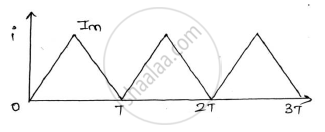# BE Production Engineering Semester 1 (FE First Year) - University of Mumbai Important Questions for Basic Electrical and Electronics Engineering

Subjects
Topics
Subjects
Popular subjects
Topics
Basic Electrical and Electronics Engineering
< prev  1 to 16 of 16  next >

State Norton’s theorem and draw the Norton’s equivalent circuit.

Appears in 1 question paper
Chapter:  D.C. Circuits
Concept: Norton’S Theorem

Using the mesh analysis find the mesh current in the direction shown and also find the voltage across A and B terminals.

Appears in 1 question paper
Chapter:  D.C. Circuits
Concept: Mesh Analysis

Find current IX using Superposition theoremAppears in 1 question paper
Chapter:  D.C. Circuits
Concept: Superposition Theorem

Determine the value of R for maximum power transfer and find the value of maximum transfer.Appears in 1 question paper
Chapter:  D.C. Circuits
Concept: Maximum Power Transfer Theorem

Find the RMS value of the waveform given below:-Appears in 1 question paper
Chapter:  A.C Circuits
Concept: RMS and Average Value

In an R-L-C parallel circuit the current through the resistor, inductor(pure), and capacitor (pure) are 20A, 15A and 40A respectively. What is the current from the supply ?draw the phasor diagram.

Appears in 1 question paper
Chapter:  A.C Circuits
Concept: R-L, R-C and R-LC parallel circuits

Prove that the average power taking by a pure capacitor fed with a sinusoidal ac supply in a circuit is zero.

Appears in 1 question paper
Chapter:  A.C Circuits
Concept: Power and Power Factor

Derive the formula for resonant frequency of the circuit with a pure capacitor in parallel with a coil having resistance and inductance. Find the expression for dynamic resistance of this parallel resonant circuit.

Appears in 1 question paper
Chapter:  A.C Circuits
Concept: Series and Parallel Resonance

A resistance and a capacitor connected in series across a 250V supply draws 5A at 50Hz. When frequency is increased to 60Hz, it draws 5.8A. Find the values of R & C. Also find active power and power factor in both cases.

Appears in 1 question paper
Chapter:  A.C Circuits
Concept: AC Through Resistance

A balanced 3 phase star connected load consists of three coils each consisting of R=6Ω and 𝑿𝑳= 8Ω . Determine the line current , power factor when the load is connected across 400V, 50Hz, supply

Appears in 1 question paper
Chapter:  Three Phase Circuits
Concept: Three Phase Current Generation

Find the node voltages 𝑽𝟏,𝑽𝟐 𝒂𝒏𝒅 𝑽𝟑 and current through 0.5Ω

Appears in 1 question paper
Chapter:  Three Phase Circuits
Concept: Three Phase Voltage Genration

Draw the phasor diagram of a single phase transformer when it is loaded with a lagging power factor load.

Appears in 1 question paper
Chapter:  Single Phase Transformer
Concept: phasor diagrams of Single Phase Transformer

A single phase transformer has 1000 turns on the primary and 200 turns on the secondary. The no load current is 3A at a power factor of 0.2 lag and the secondary current is 280A at a power factor of 0.8 lag. Neglect Rand X2 calculate (1) Magnetizing component and loss component of no load current. (2) primary current (3) input power factor .Draw phasor diagram showing all the currents.

Appears in 1 question paper
Chapter:  Single Phase Transformer
Concept: Construction of Single Phase Transformer

Describe the basic principle of operation of a single phase transformer and derive the emf equation.

Appears in 1 question paper
Chapter:  Single Phase Transformer
Concept: Working Principle of Single Phase Transformer

The OC and SC test data are given below for a single phase, 5KVA, 200V/400V, 50Hz transformer

 OC test from LV side 200V 1.25A 150w SC test from HV side 20V 12.5A 175w

Determine the following :-
1) Draw the equivalent circuit of the transformer referred to LV side.
2) At what load or KVA the transformer is to be operated for maximum efficiency?
3) Calculate the value of maximum efficiency.
4) Regulation of the transformer at full load 0.8power factor lagging.

Appears in 1 question paper
Chapter:  Single Phase Transformer
Concept: OC and SC Test

Briefly explain the classification of DC machine.

Appears in 1 question paper
Chapter:  DC Machines
Concept: Construction and Classification of Dc Machines
< prev  1 to 16 of 16  next >